# Introduction

It ’s a low-level story. It's easy to read if Java can be made normal for the time being. If you have compared strings, you can read it for the time being.

# review(?)

When comparing strings in Java, do not compare with "==". Use the String # equals method.

``````public class Test {
public static void main(String[] args) {
String a = "HelloWorld";
String b = "Hello";
b += "World";

System.out.println(a == b);        // false
System.out.println(a.equals(b));   // true
}
}
``````

# Wait a minute, "==" will be true

``````public class Test {
public static void main(String[] args) {
String a = "HelloWorld";
String b = "HelloWorld";

System.out.println(a == b);       // true
System.out.println(a.equals(b));  // true
}
}
``````

Why?

# Mechanism of "=="

JVM "==" Is often said to be a comparison of address locations, but let's take a closer look.

In Java, "==" is often converted to one of the JVM instructions, such as "if_acmpne" or "if_acmpeq", at compile time.

The JVM produces a lot of class files when you compile Java files, right? The Java Virtual Machine (JVM) interprets and executes the class file. Class files are in a format that many people can't read (and rarely do), but they're easier to read in the JVM (computer). Since the JVM realizes a complex program by combining multiple simple instructions, what is only one instruction in Java is often converted into multiple instructions in the JVM.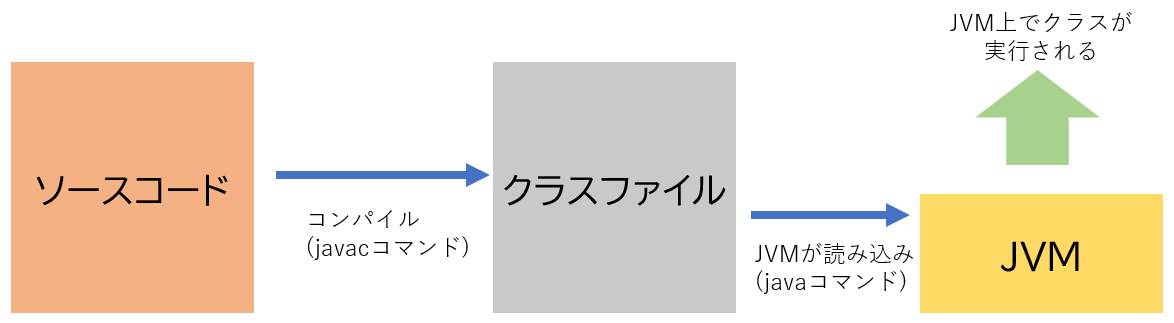## JVM instruction and operand stack

Then, what kind of instruction is "if_acmpne" or "if_acmpeq" is an instruction that takes out two from the operand stack, checks whether they match, and jumps to the line of the specified program.

Operand stack ……? The JVM is called a stack machine, and does not use registers, but uses a device called a "stack" to perform various operations.

• Stack: A device that allows you to enter values from the top and take them out in order (like a deck of playing cards)

The operand stack is like a workspace. It is often used because it is just right for four arithmetic operations. (Reverse Polish notation, etc.)

For example, 5 + 12 can be calculated by the following mechanism.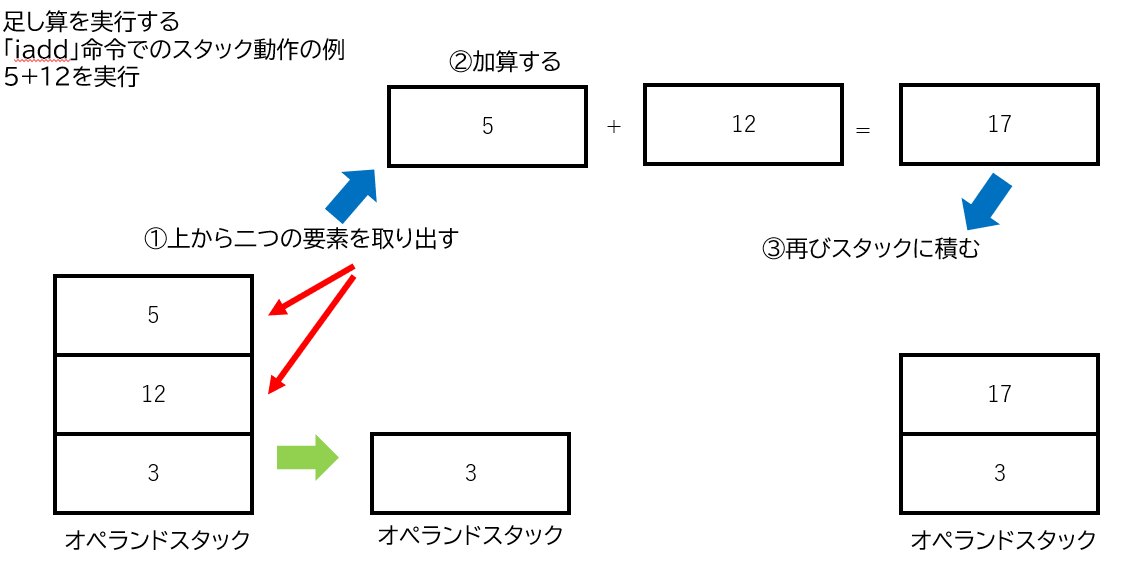However, the operand stack in Java has only one element up to 32 bits.

• It means that it can handle up to 32bit = 2 ^ 32.

When one character is represented by Java, one element (= 32bit) of this operand stack is consumed. The string is variable size. Sometimes we handle one character, and sometimes we handle hundreds of characters as in this article. In other words, one element of the operand stack (= 32bit) cannot handle all characters at all.

Therefore, save the string in a separate memory and save the address in that memory (the address on your computer) in the operand stack. As a result, one element of the operand stack (= 32bit) is sufficient.

• Image of another memory and operand stack using it (0x has no particular meaning)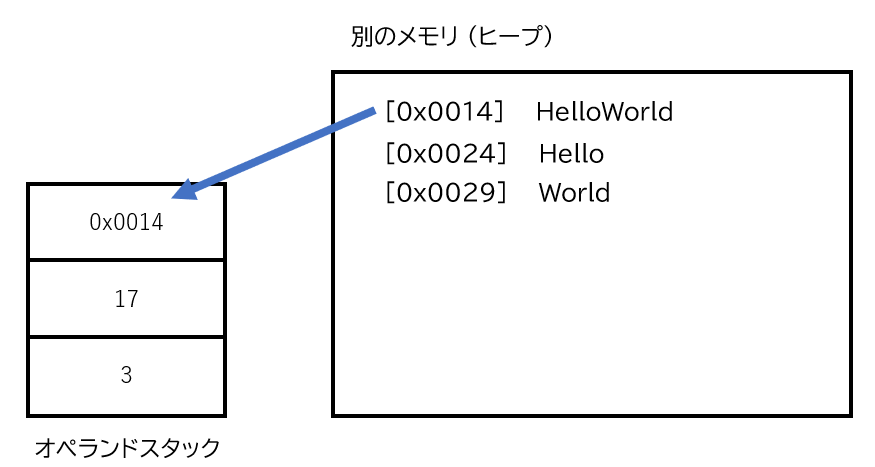if_acmpeq / if_acmpne

As mentioned earlier, "if_acmpne" and "if_acmpeq" are taken from the operand stack, and when they are equal for "if_acmpeq" and not equal for "if_acmpne", they jump to another specified line. (Jump is like a common GOTO command)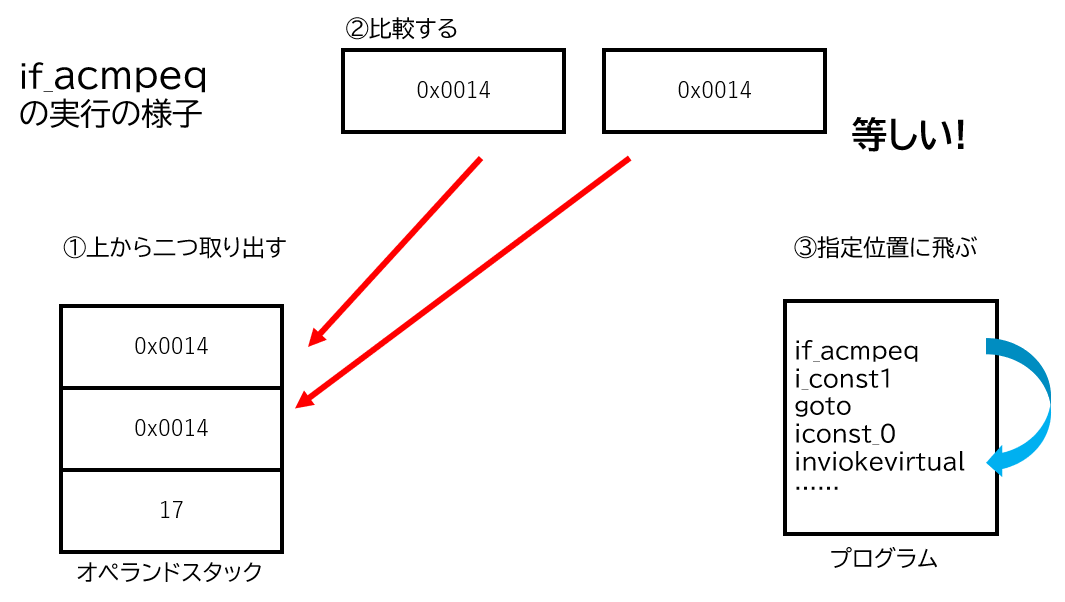In other words, with "if_acmpeq", if the numbers of the addresses on the operand stack are equal, a jump instruction will be generated. In the second source code of this page ("Wait a minute"), "Hello World" was stored at the same address, so it is displayed as true.

# To be true ...

## Is it stored in the same place?

That's it.

So what do you think this will be? Please forgive that the naming is appropriate

``````public class Test {
public static void main(String[] args) {
String a = "HelloWorld";
method1(a);
}

public static void method1(String c) {
String k = "HelloWorld";
System.out.println(k == c);
}
}
``````

## Class constant area

Java class files have a constant area. This constant area mainly stores character strings, etc. when compiling. Things like magic numbers and magic strings (?) Are stored in this constant area and are read into memory and used when the JVM reads the class file. For example

``````System.out.println("Hello Ja! Ja!");
``````

Such as "Hello Ja! Ja!" Is stored in the constant area and the character string is read from there.

Also, the Java compiler is smart enough that even if the same string appears twice, it will be read from the same constant area if it has been used more than once before. In other words, in the previous code, even if the methods were different, the addresses were the same because they referred to the string "Hello World" in the constant area of the same class.

If you look at the constant area of the class file, binary, "Hello World" is saved properly.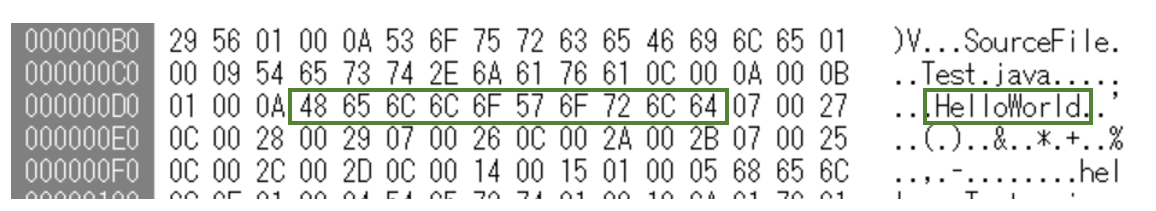As an aside, in the first source code on this page, I intentionally wrote the strings in two parts, "Hello" and "World", in order to separate the storage area. The Java compiler doesn't seem to do that much. C compilers often do this.

It is overridden by the String class, and it is a code that compares the contents firmly The image code looks like the one below. Not the original sic.

``````@Override
public boolean equals(String str) {
if(this.length() != str.length()) {
return false;
}
for(int i = 0; i < str.length(); i++) {
if(this.charAt(i) != str.charAt(i)) {    //You can retrieve any nth character with charAt
return false;
}
}
return true;
}
``````

The characters are compared one by one linearly from the front. There is no problem because one character fits in 32bit.

# Summary

Use the equals method when comparing strings. When "==" is true, it is stored in the same place. Hmmm.

The article on tomorrow's Advent calendar is also good! .. Shizuoka University Information LT Tournament Advent Calendar 2019